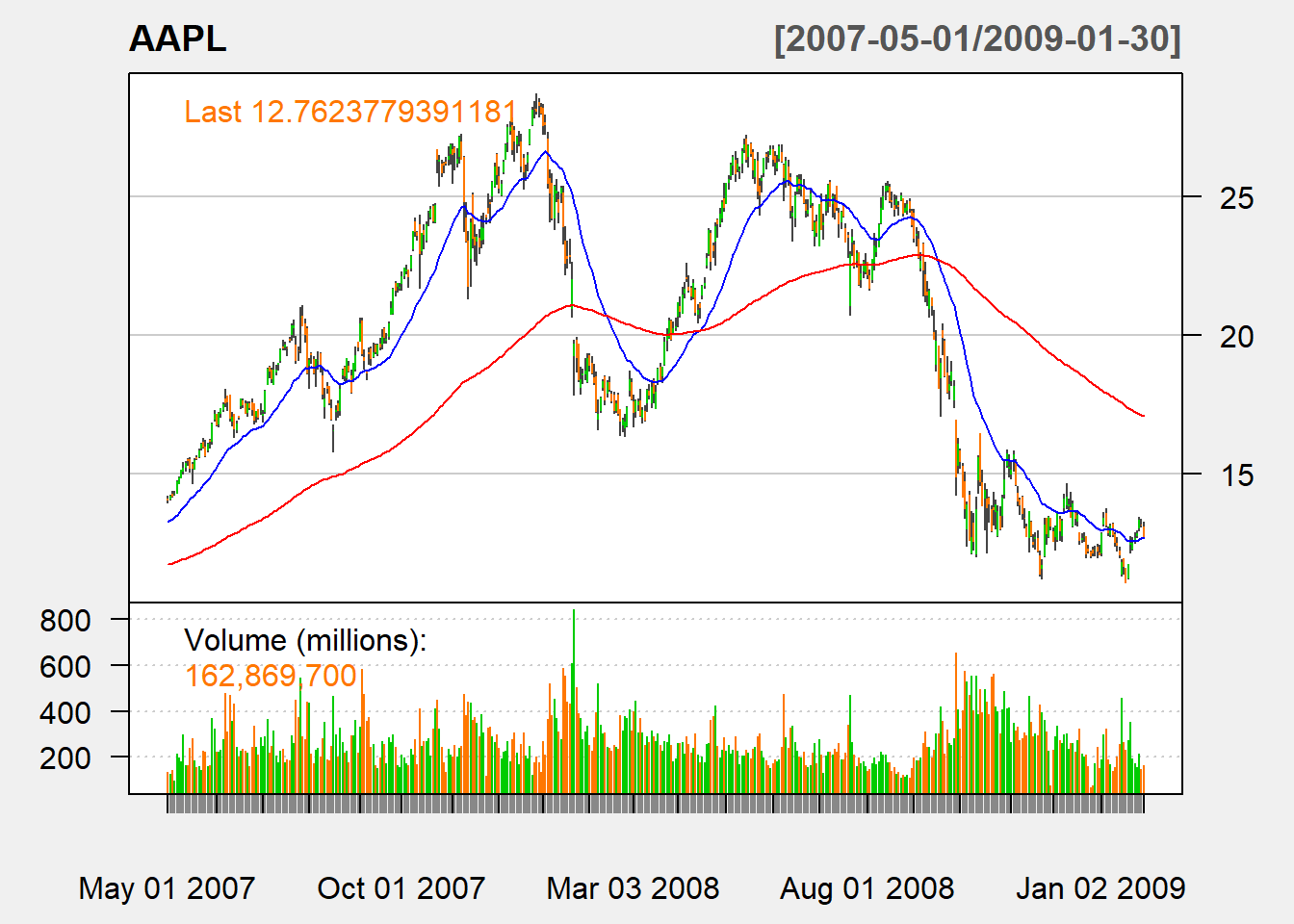## 5.3 Exponential moving average (EMA)

EMA with $$n$$ lagged period at time $$t$$: \begin{align*} &ema_t(P,n)\\ &= \beta P_t + \beta (1-\beta)P_{t-1}+\beta(1-\beta )^{2}P_{t-2}+ \cdots\\ &=\beta P_t + (1-\beta) ema_{t-1}(P,n) \end{align*}

where the smoothing coefficient $$\beta$$ is usually$\beta=\frac{2}{n+1}$

myEMA <- function (price,n){
ema <- c()
ema[1:(n-1)] <- NA
ema[n]<- mean(price[1:n])
beta <- 2/(n+1)
for (i in (n+1):length(price)){
ema[i]<-beta * price[i] +
(1-beta) * ema[i-1]
}
ema <- reclass(ema,price)
return(ema)
}

Let us apply our function:

ema <-myEMA(Cl(AAPL),n=20)
tail(ema,n=3)
##                [,1]
## 2012-12-26 76.64302
## 2012-12-27 76.35131
## 2012-12-28 76.01295

In the application, the first EMA will be given by SMA, and subsequent EMAs is calcualted by the update formula.

### 5.3.1 TTR

In the TTR package, we can use EMA():

ema <-EMA(Cl(AAPL),n=20)
tail(ema,n=3)
##                 EMA
## 2012-12-26 76.64302
## 2012-12-27 76.35131
## 2012-12-28 76.01295

We can see that our code gives the same result.

Buy signal arises when a short-run EMA crosses from below to above a long-run EMA.

Sell signal arrises when a short-run EMA crosses from above to above a long-run EMA.

### 5.3.3 Charting

chartSeries(AAPL,
subset='2007-05::2009-01',
theme=chartTheme('white'))
addEMA(n=200,on=1,col = "red")